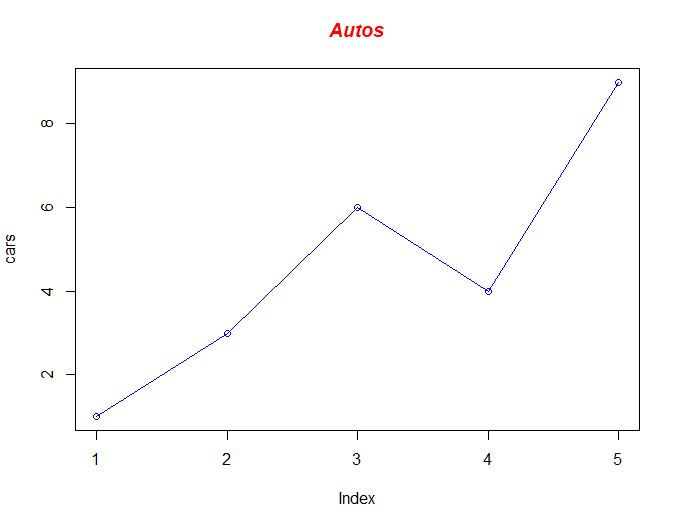top of page
Search

# R Assignment Help | Data Visualization Using R

In this post, we will learn how to draw the line graph using R Studio.

# Define the cars vector with 5 values

cars <- c(1, 3, 6, 4, 9)

# Graph the cars vector with all defaults

plot(cars)

Output:### Now joining the line using:

# Define the cars vector with 5 values

cars <- c(1, 3, 6, 4, 9)

# Graph cars using blue points overlayed by a line

plot(cars, type="o", col="blue")

# Create a title with a red, bold/italic font

title(main="Autos", col.main="red", font.main=4)

Output:### Drawing multiple lines

# Define 2 vectors

cars <- c(1, 3, 6, 4, 9)

trucks <- c(2, 5, 4, 5, 12)

# Graph cars using a y axis that ranges from 0 to 12

plot(cars, type="o", col="blue", ylim=c(0,12))

# Graph trucks with red dashed line and square points

lines(trucks, type="o", pch=22, lty=2, col="red")

# Create a title with a red, bold/italic font

title(main="Autos", col.main="red", font.main=4)### Data visualization using the legend

# Define 2 vectors

cars <- c(1, 3, 6, 4, 9)

trucks <- c(2, 5, 4, 5, 12)

# Calculate range from 0 to max value of cars and trucks

g_range <- range(0, cars, trucks)

# Graph autos using y axis that ranges from 0 to max

plot(cars, type="o", col="blue", ylim=g_range,

axes=FALSE, ann=FALSE)

# Make x axis using Mon-Fri labels

axis(1, at=1:5, lab=c("Mon","Tue","Wed","Thu","Fri"))

# Make y axis with horizontal labels that display ticks at

# every 4 marks. 4*0:g_range is equivalent to c(0,4,8,12).

axis(2, las=1, at=4*0:g_range)

# Create box around plot

box()

# Graph trucks with red dashed line and square points

lines(trucks, type="o", pch=22, lty=2, col="red")

# Create a title with a red, bold/italic font

title(main="Autos", col.main="red", font.main=4)

# Label the x and y axes with dark green text

title(xlab="Days", col.lab=rgb(0,0.5,0))

title(ylab="Total", col.lab=rgb(0,0.5,0))

# Create a legend at (1, g_range) that is slightly smaller

# (cex) and uses the same line colors and points used by

# the actual plots

legend(1, g_range, c("cars","trucks"), cex=0.8,

col=c("blue","red"), pch=21:22, lty=1:2);

Output:Thanks for reading if you need any types of data visualization help like pie graph, bar graph, etc, you can contact us at given below link

Get your project or assignment completed by R expert and experienced developers and researchers.

OR

If you have project files, You can send at codersarts@gmail.com directly